##### Common Core Math For Parents For Dummies with Videos Online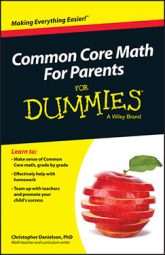Being able to solve proportions is important in seventh grade math. In a Common Core classroom, the emphasis is on making sense of the solution method, rather than on memorizing a given procedure.

One general rule for whether your child is making sense of his solution is this: Does he know what each number means along the way?

Consider this question: you ran 5 miles in 40 minutes yesterday. If you run at the same rate, do you have time for a 3‐mile run right now? In order to answer this question, a student could figure out the time it should take him to run 3 miles. He might write this proportion: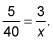In the spirit of keeping track of the meaning of numbers as you work, here are two ways a typical seventh grader might solve this proportion. In each case, you look at what meanings the student might have for the numbers.

• Unit rate: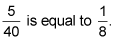This means that you run 1 mile every 8 minutes (on average) if you ran 5 miles in 40 minutes. One mile every 8 minutes is a unit rate. Using this unit rate, you must run 3 miles in 24 minutes.

In this solution, every number has a meaning. The fraction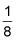is a rate that compares miles to minutes. You can think of this as "1 mile for every 8 minutes" or as "one‐eighth of a mile per minute." Either way, you can multiply 8 by 3 because running 3 miles takes 3 times as long as running 1 mile. Because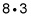is 24, it should take 24 minutes to run three miles. This strategy builds on strategies for working with equivalent fractions and ratios, which are already familiar to students.

• Cross‐multiply and divide: Multiplying 40 by 3 gives 120. (This is the cross‐multiplication part.) Dividing 120 by 5 gives 24, so you can run 3 miles in 24 minutes.

In this solution, it's not at all obvious what the 120 means in the context of running. Forty minutes times 3 miles equals 120‐minute miles? This doesn't make sense. And then why divide 120 by 5? Without a meaning for the 120, it's hard to say why you're dividing.

A typical seventh grader can work out the first solution, given time to think. This solution makes sense, and it's based on other things that he knows well. A seventh grader would be unlikely to come up with the second solution alone.

In a Common Core classroom, teachers encourage students to develop strategies, such as the first solution, rather than telling students solution methods that don't make sense to them.